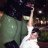# Request for MACD Divergence Scanner Change to True Divergence

M

#### me waste xp

##### New member
Hey guys.

I'd like to change the MACD Divergence scanner (the one with the YouTube video on it: video here.) to scan for only "true" divergences.

To illustrate, let's take a bullish divergence, I mean that I want the scanner to only give me charts for which a rally/positive MACD occurs between the two divergence prices.

Here's the code of interest:

Code:
``````# MACD Divergence Pivots
# Mobius
# V03.05.2015 Upper Study

input n = 2;

input fastLength = 12;

input slowLength = 26;

input MACDLength = 9;

input averageType = AverageType.EXPONENTIAL;

def h = high;

def l = low;

def bar = barNumber();

def Diff = MACD(fastLength, slowLength, MACDLength, averageType).Diff;

def CurrMACDh = if Diff > 0

then fold i = 1 to n + 1

with p = 1

while p

do Diff > getValue(Diff, -i)

else 0;

def CurrMACDPivotH = if (bar > n and

Diff == highest(Diff, n) and

CurrMACDh)

then h

else double.NaN;

def CurrMACDl = if Diff < 0

then fold j = 1 to n + 1

with q = 1

while q

do Diff < getValue(Diff, -j)

else 0;

def CurrMACDPivotL = if (bar > n and

Diff == lowest(Diff, n) and

CurrMACDl)

then l

else double.NaN;

def CurrPHBar = if !isNaN(CurrMACDPivotH)

then bar

else CurrPHBar;

def CurrPLBar = if !isNaN(CurrMACDPivotL)

then bar

else CurrPLBar;

def PHpoint = if !isNaN(CurrMACDPivotH)

then CurrMACDPivotH

else PHpoint;

def priorPHBar = if PHpoint != PHpoint

then CurrPHBar

else priorPHBar;

def PLpoint = if !isNaN(CurrMACDPivotL)

then CurrMACDPivotL

else PLpoint;

def priorPLBar = if PLpoint != PLpoint

then CurrPLBar

else priorPLBar;

def HighPivots = bar >= highestAll(priorPHBar);

def LowPivots = bar >= highestAll(priorPLBar);

def pivotHigh = if HighPivots

then CurrMACDPivotH

else double.NaN;

plot PlotHline = pivotHigh;

PlotHline.enableApproximation();

PlotHline.SetDefaultColor(GetColor(7));

PlotHline.SetStyle(Curve.Short_DASH);

plot pivotLow = if LowPivots

then CurrMACDPivotL

else double.NaN;

pivotLow.enableApproximation();

pivotLow.SetDefaultColor(GetColor(7));

pivotLow.SetStyle(Curve.Short_DASH);

plot PivotDot = if !isNaN(pivotHigh)

then pivotHigh

else if !isNaN(pivotLow)

then pivotLow

else double.NaN;

pivotDot.SetDefaultColor(GetColor(7));

pivotDot.SetPaintingStrategy(PaintingStrategy.POINTS);

pivotDot.SetLineWeight(3);

# End Code Pivots with Projections``````
If you could point me in the right direction to learn to do this myself, I'd appreciate that, too.

I just don't know how to interpret, let alone edit this code. #LearnToCode?

M

#### me waste xp

##### New member
What if I add a payment to the request? A few bucks?Questions 6